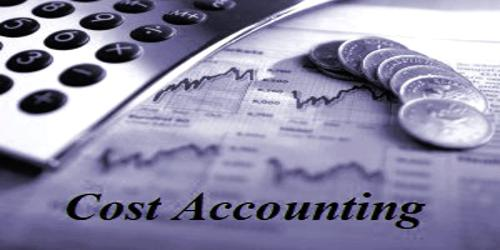Accounting

# Cost Accounting CycleCost Accounting Cycle

Cost accounting is the process of collecting information about the costs incurred by a company’s activities, assigning selected costs to products and services and other cost objects, and evaluating the efficiency of cost usage.

Cost Accounting cycle is a process performed during the accounting period in recording data, classifying, determining total cost, determining product cost, determining the selling price, controlling cost and decision making.

(a) Recording cost data: At the first step of cost accounting it ascertains and records the element of cost for determining of the cost of production.

(b) Classification of cost: At the Second step according to function, nature, and behavior cost accounting classifies the cost.

(c) Determining total cost: In this step under cost accounting cost of goods sold of a product is calculated. Cost of goods sold means the sum of all items of expenditure incurred in production for the goods which are sold.

(d) Unit cost: At the fourth step Unit cost is obtained dividing the cost of goods sold divided by a total number of unit sold.

(e) Selling price: Selling price is obtained by adding a profit margin with the cost of sales.

(f) Cost Control and decision making: At the last step of cost accounting by using standard costing and budget and budgetary control system cost accounting control cost and make a decision.